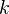# Milnor K-theory

<]]>

<]]>

<]]>
• ## Paper References

Chapter 7 of Gille and Szamuely (in Various folder under ALGEBRA)

<]]>

<]]>

<]]>
• ## Standard theorems

The Milnor conjecture. See for example Kahn's Bourbaki account, reproduced in K-theory handbook, Vol 2.

<]]>

<]]>

<]]>

<]]>

<]]>
• ## Some Research Articles

Akhtar

Thomason: Le principe de scindage. He shows roughly that any theory for schemes satisfying certain axioms and mapping to Quillen K-theory, must be surjective when evaluated on the ground field. This implies that Milnor K-theory, if required to satisfy these axioms, cannot be extended to the category of varieties over.

Kerr: A regulator formula for Milnor K-groups (2003)

Motivic interpretation of Milnor K-groups attached to Jacobian varieties, by Satoshi Mochizuki: K0774

Müller-Stach and Elbaz-Vincent: Milnor K-theory of rings, higher Chow groups and applications

The Gersten conjecture for Milnor K-theory , by Moritz Kerz

MR2034645 (2004m:19003) Cathelineau, Jean-Louis(F-NICE-LD) Projective configurations, homology of orthogonal groups, and Milnor \$K\$-theory.

Milnor: Algebraic K-theory and quadratic forms (1969/70)

Totaro: Milnor K-theory is the simplest part of algebraic K-theory (1992)

Voevodsky, Vishik, Orlov: An exact sequence for mod 2 Milnor K-th etc. Contains an exact seq for the multiplication in mod 2 Milnor Kth with an element, and applications to questions on quadratic forms, e.g. the Milnor conjecture and the J-filtration conjecture.

<]]>

<]]>